• 刚体上速度
2021-06-16 11:48:22

unity中c#脚本语言中偶尔要判断数字键的输入；

给予刚体各个方向的速度；

首先要给予物体刚体部件Rigidbody；

public Rigidbody2D rb;

rb为一个物体的刚体部件；

rb.velocity = new Vector2(horizontalmove * speed, rb.velocity.y);

这样就设置了rb的x方向的速度；

当然这个是2d的物体；

3d这个类似；unity
更多相关内容
• 本文目录1 旋转速度2 刚体速度2.1 定义2.2 伴随变换3 旋量运动的速度 科学家必须在庞杂的经验事实中抓住某些可用精密公式来表示的普遍特征，由此探求自然界的普遍原理。 ——爱因斯坦 1 旋转速度 先考虑 R3\R^3R3 中...
• 4. 回转刚体上任意一点速度刚体角速度的关系。上面x不是表示矩阵的简单相乘，而是叉乘。

1. 二维平面做圆周的质点速度与角速度关系：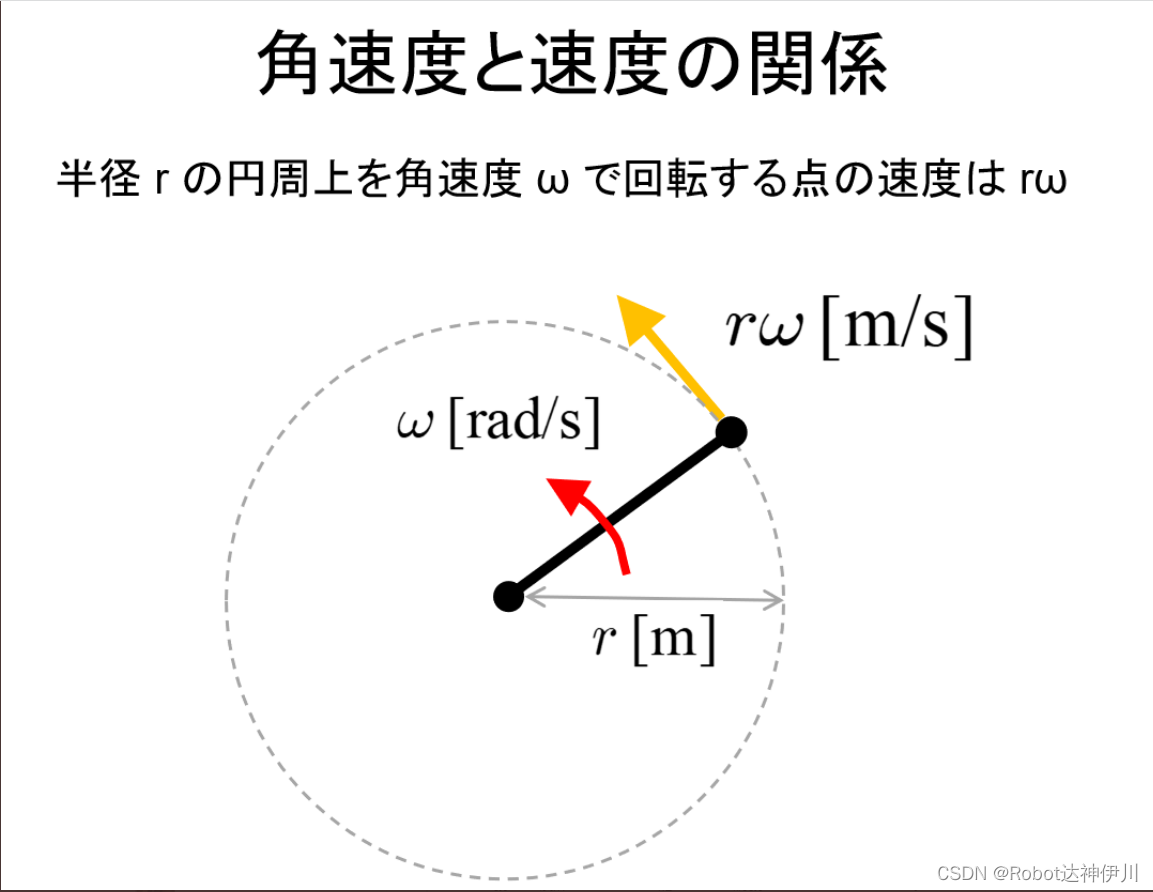2. 点的速度x,y方向分解：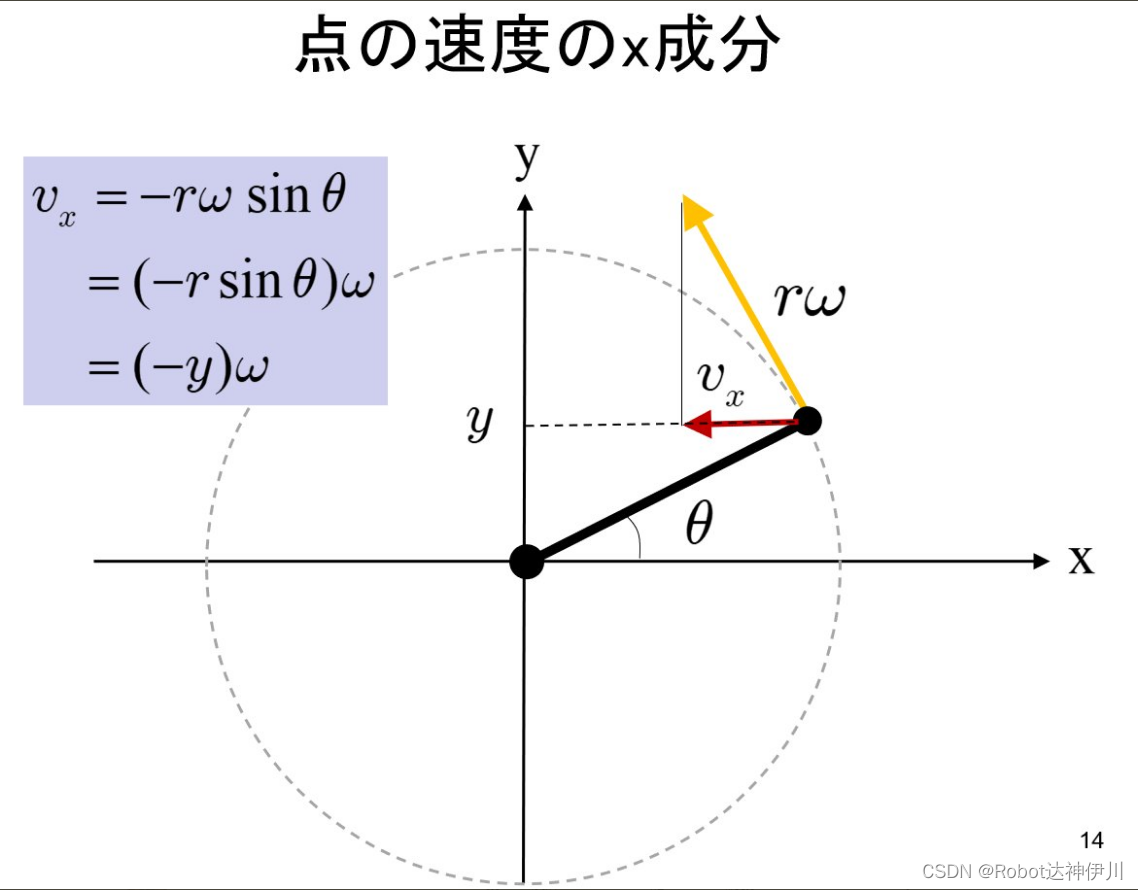3.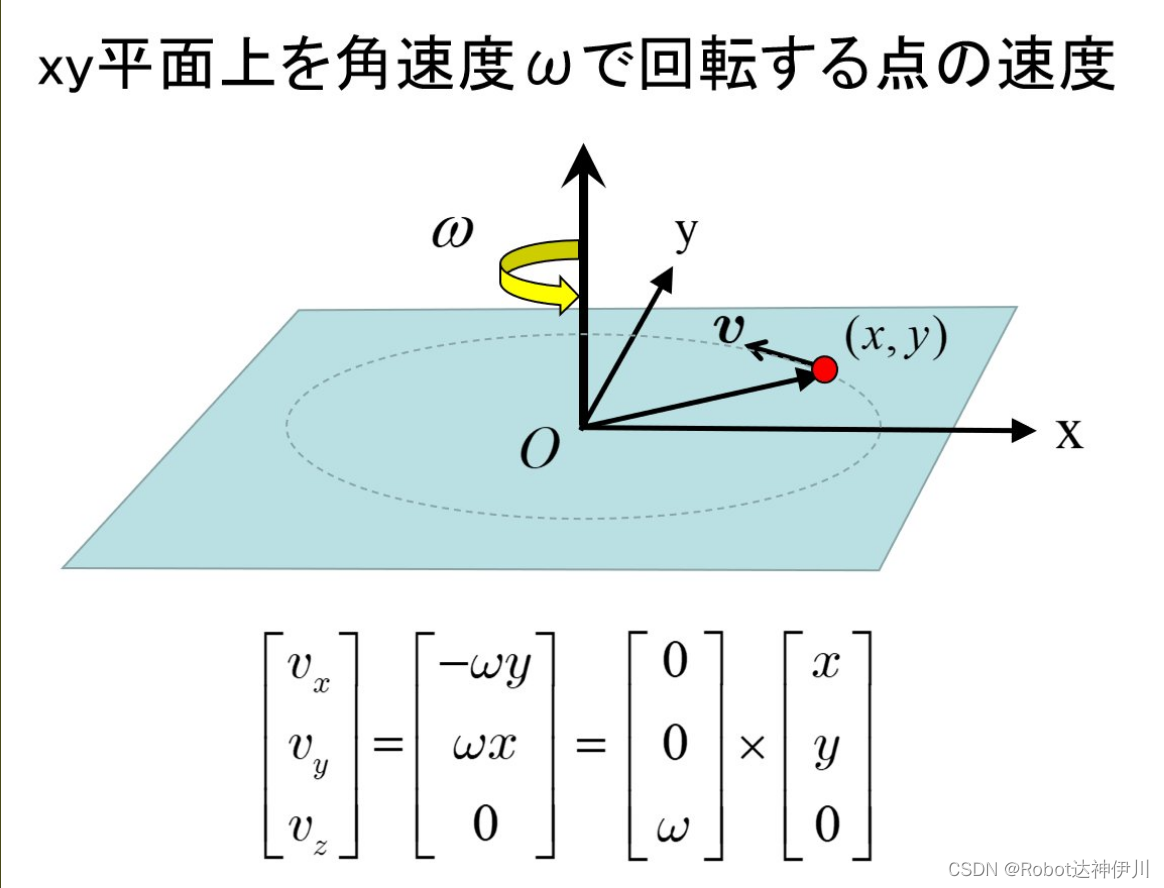上面x不是表示矩阵的简单相乘，而是叉乘。

4. 回转刚体上任意一点速度与刚体角速度的关系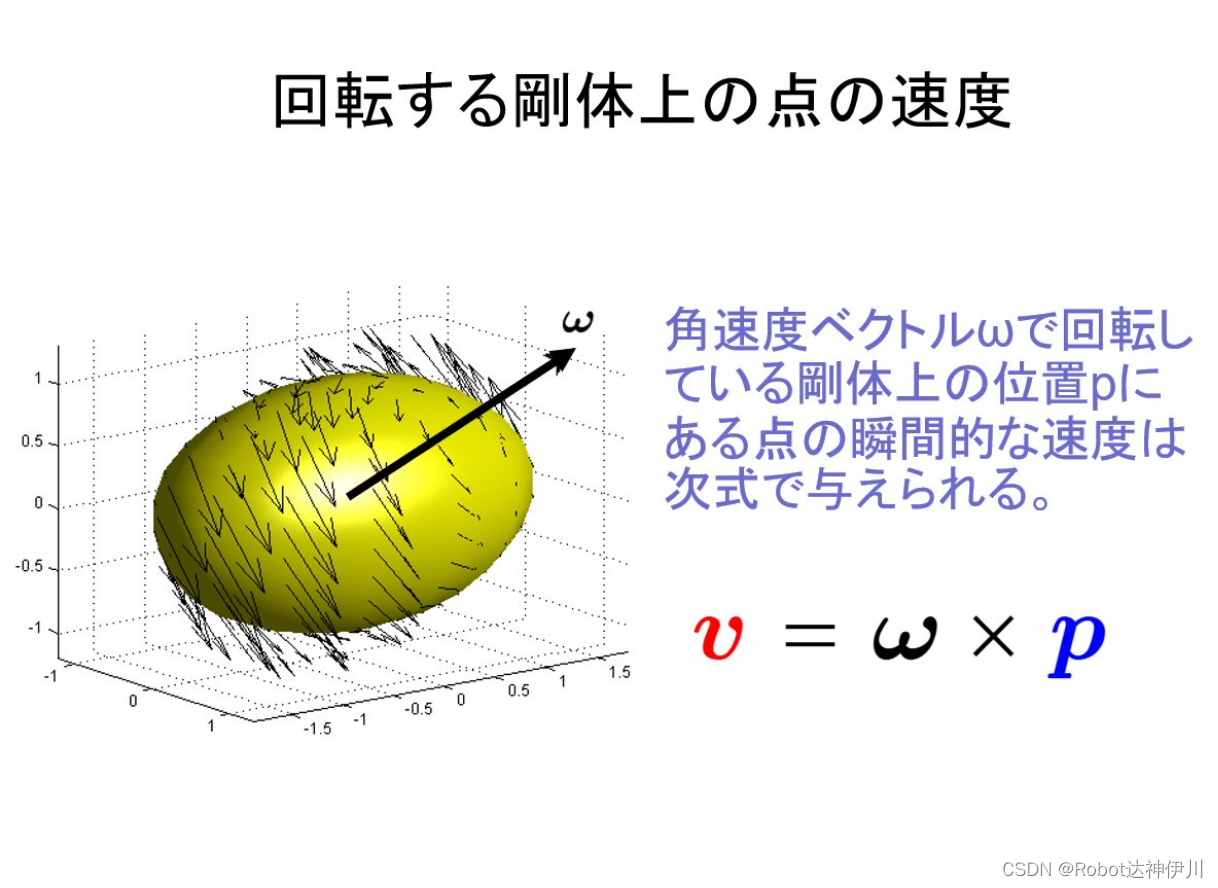Reference:

展开全文• 刚体速度只有在选定坐标系时才有谈论意义，比如一台小车可以看作是一个刚体，其有三个传感器，Camera有一个速度，IMU有一个速度，轮子有一个速度，首先它们的速度是不同的（比如把地球看作一个刚体，地心的速度...

参考：《A Mathematical Introduction to Robotic Manipulation》

疑问：

• 刚体的速度只有在选定坐标系时才有谈论意义，比如一台小车可以看作是一个刚体，其上有三个传感器，Camera有一个速度，IMU有一个速度，轮子有一个速度，首先它们的速度是不同的（比如把地球看作一个刚体，地心的速度与地球表面的速度是不同的），那么这些速度的关系是什么呢？
• VIO中为何不使用Camera系而是IMU作为body系，如果使用Camera作为body系，IMU的测量值（角速度和加速度）要如何转化到Camera坐标系下？

#### 1. 转动速度

刚体上任意一点 q q 在空间坐标系中的运动轨迹为：
q a ( t ) = R a b ( t ) q b \mathbf{q}_{a}(t)=\mathbf{R}_{a b}(t) \mathbf{q}_{b}

其中，A为空间坐标系，B为物体坐标系，坐标 q b \mathbf{q}_b 是在物体坐标系中固定不变的，该点在空间坐标系中的速度为：
v q a ( t ) = d d t q a ( t ) = R ˙ a b ( t ) q b \mathbf{v}_{\mathbf{q}_{a}}(t)=\frac{d}{d t} \mathbf{q}_{a}(t)=\dot{\mathbf{R}}_{a b}(t) \mathbf{q}_{b}

因此， R a b \mathbf{R}_{a b} 将一点的刚体坐标变换为该点的空间速度，类似 R a b \mathbf{R}_{a b} 这种表示需要九个参数，不是很方便，转换成下面的简洁形式：
v q a ( t ) = R ˙ a b ( t ) R a b − 1 ( t ) R a b ( t ) q b v_{q_{a}}(t)=\dot{R}_{a b}(t) R_{a b}^{-1}(t) R_{a b}(t) q_{b}

我们已经知道 R ˙ a b ( t ) R a b − 1 ( t ) ∈ so ⁡ ( 3 ) \dot{R}_{a b}(t) R_{a b}^{-1}(t) \in \operatorname{so}(3) ，是反对陈矩阵，记为：
ω ^ a b s : = R ˙ a b R a b − 1 \widehat{\omega}_{a b}^{s}:=\dot{R}_{a b} R_{a b}^{-1}

其中， ω ^ a b s \widehat{\omega}_{a b}^{s} 表示 b b 系在 a a 系下的速度在空间坐标系下的表示，描述为物体相对空间坐标系A的瞬时角速度

##### 1.1 刚体上一点的速度（A系与B系之间只有纯旋转运动）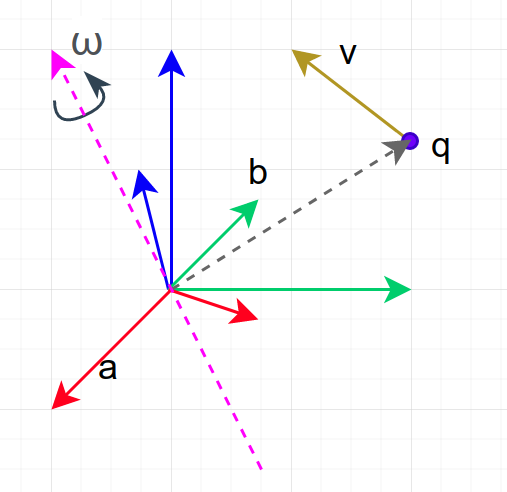现在就可以使用物体的瞬时角速度来表示一点的速度，如下：
v q a ( t ) = ω ^ a b s R a b ( t ) q b = ω a b s ( t ) × q a ( t ) v_{q_{a}}(t)=\widehat{\omega}_{a b}^{s} R_{a b}(t) q_{b}=\omega_{a b}^{s}(t) \times q_{a}(t)

对应的物理含义为：刚体的转速与刚体上一点到坐标系原点的距离的叉乘即为这一点的瞬时速度， q b q_{b} 这个向量的长度越长，速度就越大，反之亦然，当长度为0时（该点与坐标系原点重合时），速度为0

这样，只要知道物体的瞬时角速度和物体坐标上任意一点的位置，就可以得到该点在空间坐标下的速度

##### 1.2 （瞬时）物体坐标系下的转动速度

同理也可得到 ω ^ a b b \widehat{\omega}_{a b}^{b} ，如下：
ω ^ a b b : = R a b − 1 R ˙ a b \widehat{\omega}_{a b}^{b}:=R_{a b}^{-1} \dot{R}_{a b}

它描述的是相对于瞬时物体坐标系B的角速度（ b b 系在 a a 系下的速度在物体坐标系下的表示），该角速度可以直接通过陀螺仪直接测量得到

由以上两式可得两种角速度之间的关系：
ω ^ a b b = R a b − 1 ω ^ a b s R a b  or  ω a b b = R a b − 1 ω a b s \widehat{\omega}_{a b}^{b}=R_{a b}^{-1} \widehat{\omega}_{a b}^{s} R_{a b} \quad \text { or } \quad \omega_{a b}^{b}=R_{a b}^{-1} \omega_{a b}^{s}

因此，物体角速度为将空间角速度旋转到瞬时物体坐标系而得到的

#### 2. 刚体速度

对于运动轨迹为为单参数曲线 g a b ( t ) ∈ S E ( 3 ) g_{a b}(t) \in S E(3) 的一般情况
g a b ( t ) = [ R a b ( t ) p a b ( t ) 0 1 ] g_{a b}(t)=\left[\begin{array}{cc} R_{a b}(t) & p_{a b}(t) \\ 0 & 1 \end{array}\right]

可得，
g ˙ a b g a b − 1 = [ R ˙ a b p ˙ a b 0 0 ] [ R a b T − R a b T p a b 0 1 ] = [ R ˙ a b R a b T − R ˙ a b R a b T p a b + p ˙ a b 0 0 ] \dot{g}_{a b} g_{a b}^{-1}=\left[\begin{array}{cc} \dot{R}_{a b} & \dot{p}_{a b} \\ 0 & 0 \end{array}\right]\left[\begin{array}{cc} R_{a b}^{T} & -R_{a b}^{T} p_{a b} \\ 0 & 1 \end{array}\right]=\left[\begin{array}{cc} \dot{R}_{a b} R_{a b}^{T} & -\dot{R}_{a b} R_{a b}^{T} p_{a b}+\dot{p}_{a b} \\ 0 & 0 \end{array}\right]

定义 V a b s ∈ s e ( 3 ) V_{ab}^s \in se(3) 表示 b b 系在 a a 系下的速度在空间坐标系下的表示
V ^ a b s = g ˙ a b g a b − 1 , V a b s = [ v a b s ω a b s ] = [ − R ˙ a b R a b T p a b + p ˙ a b ( R ˙ a b R a b T ) ∨ ] \widehat{V}_{a b}^{s}=\dot{g}_{a b} g_{a b}^{-1} ,\quad V_{a b}^{s}=\left[\begin{array}{c} v_{a b}^{s} \\ \omega_{a b}^{s} \end{array}\right]=\left[\begin{array}{c} -\dot{R}_{a b} R_{a b}^{T} p_{a b}+\dot{p}_{a b} \\ \left(\dot{R}_{a b} R_{a b}^{T}\right)^{\vee} \end{array}\right]

虽然 V a b s ∈ s e ( 3 ) V_{ab}^s \in se(3) ，但这里的移动分量 v a b s v_{a b}^{s} 却是以m/s为单位的平移速度，因为 p ˙ a b \dot{p}_{a b} 的单位是m/s

注意，移动分量 v a b s ( t ) v_{ab}^s(t) 并不是刚体坐标系原点的速度，而是刚体上一点在 t t 时刻经过空间坐标系原点时（可能是想象的）的速度。即，如果一个人站在空间坐标系的原点处去测量刚体上正好经过原点的一点的瞬时速度，那么该瞬时速度即为 v a b s ( t ) v_{ab}^s(t)

##### 2.1 刚体上一点的速度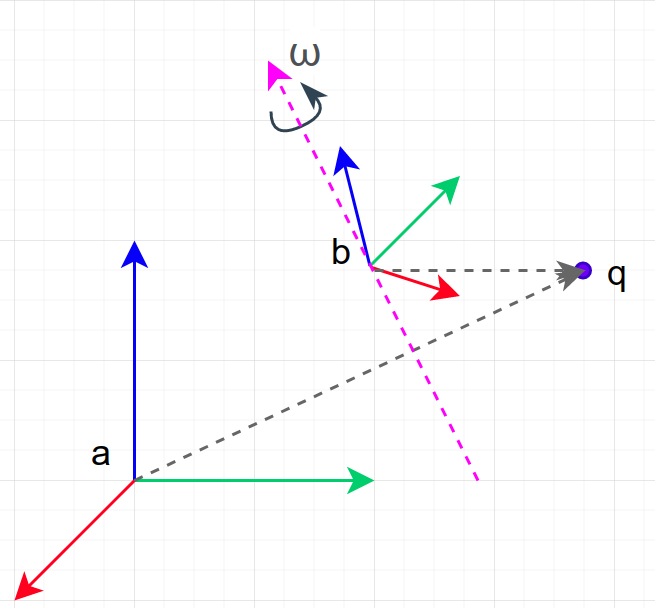在空间坐标系中，固联在刚体上的一点 q q 的坐标为
q a ( t ) = g a b ( t ) q b q_{a}(t)=g_{a b}(t) q_{b}

两边微分得
v q a = q ˙ a = g ˙ a b q b = g ˙ a b g a b − 1 q a v_{q_{a}}=\dot{q}_{a}=\dot{g}_{a b} q_{b}=\dot{g}_{a b} g_{a b}^{-1} q_{a}

进一步得到
v q a = V ^ a b s q a = ω a b s × q a + v a b s v_{q_{a}}=\widehat{V}_{a b}^{s} q_{a}=\omega_{a b}^{s} \times q_{a}+v_{a b}^{s}

其中 v q a v_{q_{a}} 由两部分构成， ω a b s × q a \omega_{a b}^{s} \times q_{a} 表示因旋转产生的速度， v a b s v_{a b}^{s} 表示平移产生的速度，两个向量相加即为最终的合成速度

##### 2.2 （瞬时）物体坐标系下的刚体速度

向量可以直接通过坐标系之间旋转外参进行转换

由于 v q a v_{q_{a}} 是向量，使用旋转矩阵进行转换，可得物体坐标系中一点的速度为：
[ v q b 0 ] = g a b − 1 [ v q a 0 ] \begin{bmatrix} v_{q_{b}} \\ 0 \end{bmatrix}=g_{a b}^{-1} \begin{bmatrix} v_{q_{a}} \\ 0 \end{bmatrix}

忽略矩阵维度，简写成：
v q b = g a b − 1 v q a = g a b − 1 g ˙ a b q b = V ^ a b b ( t ) q b v_{q_{b}}=g_{a b}^{-1} v_{q_{a}}=g_{a b}^{-1} \dot{g}_{a b} q_{b}=\widehat{V}_{a b}^{b}(t) q_{b}

物体坐标系下的刚体速度定义为：
V ^ a b b = g a b − 1 g ˙ a b = [ R a b T R ˙ a b R a b T p ˙ a b 0 0 ] , V a b b = [ v a b b ω a b b ] = [ R a b T p ˙ a b ( R a b T R ˙ a b ) ∨ ] \widehat{V}_{a b}^{b}=g_{a b}^{-1} \dot{g}_{a b}=\left[\begin{array}{cc} R_{a b}^{T} \dot{R}_{a b} & R_{a b}^{T} \dot{p}_{a b} \\ 0 & 0 \end{array}\right] ,\quad V_{a b}^{b}=\left[\begin{array}{c} v_{a b}^{b} \\ \omega_{a b}^{b} \end{array}\right]=\left[\begin{array}{c} R_{a b}^{T} \dot{p}_{a b} \\ \left(R_{a b}^{T} \dot{R}_{a b}\right)^{\vee} \end{array}\right]

需要指出的是，物体速度并不是物体相对于物体坐标系的速度（此速度总是零

##### 2.3 空间坐标下的刚体速度与（瞬时）物体坐标系下的刚体速度的关系

V ^ a b s = g ˙ a b g a b − 1 = g a b ( g a b − 1 g ˙ a b ) g a b − 1 = g a b V ^ a b b g a b − 1 \widehat{V}_{a b}^{s}=\dot{g}_{a b} g_{a b}^{-1}=g_{a b}\left(g_{a b}^{-1} \dot{g}_{a b}\right) g_{a b}^{-1}=g_{a b} \widehat{V}_{a b}^{b} g_{a b}^{-1}

可得，
V a b s = [ v a b s ω a b s ] = [ R a b p ^ a b R a b 0 R a b ] [ v a b b ω a b b ] = A d ( g a b ) V a b b V_{a b}^{s}=\left[\begin{array}{c} v_{a b}^{s} \\ \omega_{a b}^{s} \end{array}\right]=\left[\begin{array}{cc} R_{a b} & \widehat{p}_{a b} R_{a b} \\ 0 & R_{a b} \end{array}\right]\left[\begin{array}{c} v_{a b}^{b} \\ \omega_{a b}^{b} \end{array}\right] = \mathbf{Ad}(g_{ab}) V_{a b}^{b}

#### 3. 刚体速度的坐标转换

假如知道了IMU与底盘坐标系之间的外参以及IMU的速度，如何得到底盘坐标系的速度

##### 3.1 空间坐标系下的转换

假设存在A、B、C三个坐标系，它们在空间坐标系下的刚体速度存在下述关系：
V ^ a c s = g ˙ a c g a c − 1 = ( g ˙ a b g b c + g a b g ˙ b c ) ( g b c − 1 g a b − 1 ) = g ˙ a b g a b − 1 + g a b ( g ˙ b c g b c − 1 ) g a b − 1 = V ^ a b s + g a b V ^ b c b g a b − 1 \begin{aligned} \widehat{V}_{a c}^{s} &=\dot{g}_{a c} g_{a c}^{-1} \\ &=\left(\dot{g}_{a b} g_{b c}+g_{a b} \dot{g}_{b c}\right)\left(g_{b c}^{-1} g_{a b}^{-1}\right) \\ &=\dot{g}_{a b} g_{a b}^{-1}+g_{a b}\left(\dot{g}_{b c} g_{b c}^{-1}\right) g_{a b}^{-1} \\ &=\widehat{V}_{a b}^{s}+g_{a b} \widehat{V}_{b c}^{b} g_{a b}^{-1} \\ \end{aligned}

注意，这里使用的是 V ^ b c b \widehat{V}_{b c}^{b} ，而书里面使用的是 V ^ b c s \widehat{V}_{b c}^{s} ，好像是书里写的有问题

转换成向量的形式，
V a c s = V a b s + A d ( g a b ) V b c b {V}_{a c}^{s} = {V}_{a b}^{s} + \mathbf{Ad}(g_{a b}) {V}_{b c}^{b}

如果 g b c g_{bc} 是常量，相当于刚体上两个坐标系B和C之间的外参，此时二者的速度关系为
V a c s = V a b s {V}_{a c}^{s} = {V}_{a b}^{s}

由于两个坐标系B和C同属于一个刚体，所以两个坐标系B和C在空间坐标系下的刚体速度是相同的

将上式转换到物体坐标系
A d ( g a c ) V a c c = A d ( g a b ) V a b s V a c c = A d ( g a c ) − 1 A d ( g a b ) V a b s V a c c = A d ( g c b ) V a b s \begin{aligned} \mathbf{Ad}(g_{ac}){V}_{a c}^{c} = \mathbf{Ad}(g_{ab}) {V}_{a b}^{s} \\ {V}_{a c}^{c} = \mathbf{Ad}(g_{ac})^{-1} \mathbf{Ad}(g_{ab}) {V}_{a b}^{s} \\ {V}_{a c}^{c} = \mathbf{Ad}(g_{cb}) {V}_{a b}^{s} \end{aligned}

即，刚体上两个坐标系B和C在各自的物体坐标系下的刚体速度关系

##### 3.2 物体坐标系下的转换

同理可得，
V ^ a c c = g a c − 1 g ˙ a c = ( g b c − 1 g a b − 1 ) ( g ˙ a b g b c + g a b g ˙ b c ) = g b c − 1 g a b − 1 g ˙ a b g b c + g b c − 1 g ˙ b c = g b c − 1 V ^ a b b g b c + V ^ b c c \begin{aligned} \widehat{V}_{a c}^{c} &=g_{a c}^{-1} \dot{g}_{a c} \\ &=\left(g_{b c}^{-1} g_{a b}^{-1}\right) \left(\dot{g}_{a b} g_{b c}+g_{a b} \dot{g}_{b c}\right) \\ &= g_{b c}^{-1} g_{a b}^{-1} \dot{g}_{a b} g_{b c} + g_{b c}^{-1} \dot{g}_{b c} \\ &= g_{b c}^{-1} \widehat{V}_{a b}^{b} g_{b c} + \widehat{V}_{b c}^{c} \end{aligned}

转换成向量的形式，
V a c c = A d ( g b c − 1 ) V a b b + V b c c {V}_{a c}^{c} = \mathbf{Ad}(g_{bc}^{-1}) {V}_{ab}^{b} + {V}_{bc}^{c}

如果 g b c g_{bc} 是常量，相当于刚体上两个坐标系B和C之间的外参，此时二者的速度关系为
V a c c = A d ( g b c − 1 ) V a b b {V}_{a c}^{c} = \mathbf{Ad}(g_{bc}^{-1}) {V}_{ab}^{b}

@leatherwang
二零二零年九月二十日

展开全文• 文章目录 李群、李代数与旋转运动 空间刚体运动与Twist Twist理论入门 Twist 的螺旋解读 空间刚体运动的指数坐标表示 力、力矩与Wrench 李群、李代数与旋转运动 这里仅简单总结下李群的旋转变换等基础知识，更...

对于用欧式距离不便衡量的距离，如两个方向的距离，我们需要定义一种Counterpart，以方便统计分析。我们假设读者对于李群（Lie group）和李代数（Lie algebra）有一定了解。读者也可以参考此文。本文的符号系统均尽可能按照参考文献1、2。

## 李群、李代数与旋转运动

这里仅简单总结下李群上的旋转变换等基础知识，更详细的内容请参考此文
定义域为李群的旋转矩阵 R ∈ S O ( 3 ) R\in SO(3) 满足 R ⊤ R = I R^{\top}R = I det ⁡ R = 1 \det R = 1 。旋转矩阵可用来表示一个方向、坐标系的变换以及一个旋转过程。下文中，我们用 R a b R_{ab} 表示在坐标系 a a 下，坐标系 b b 的方向。
如下图，定义一个角速度为 ω = ω ^ θ ˙ \omega = \hat{\omega}\dot{\theta} 其中 ω ^ \hat{\omega} 为单位长度的向量，此处即旋转轴， θ ˙ \dot{\theta} 为单位时间的旋转角度。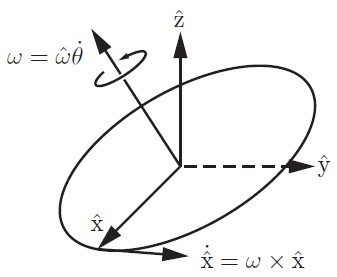那么，我们可以一次计算出空间中绕该轴以该角速度旋转的某一点的速度为 x ^ ˙ = ω × x ^ \dot{\hat{x}} = \omega \times \hat{x} 到目前我们还未指定坐标系，下述内容中，我们定义固定坐标系 s s 和刚体（随动）坐标系 b b
首先讨论坐标系 s s 下的旋转问题。在该坐标系下，令 R ( t ) ≜ R s b ( t ) R(t) \triangleq R_{sb}(t) 表示某一时刻刚体坐标系在固定坐标系下的方向，显然此时
R = [ x ^ , y ^ , z ^ ] R = [\hat{x}, \hat{y}, \hat{z}] 那么 R ˙ = [ x ^ ˙ , y ^ ˙ , z ^ ˙ ] = [ ω s × x ^ , ω s × y ^ , ω s × z ^ ] = ω s × R ≜ ω s ∧ R \dot{R} = [\dot{\hat{x}}, \dot{\hat{y}}, \dot{\hat{z}}] = [ \omega_{s} \times \hat{x}, \omega_{s} \times \hat{y}, \omega_{s} \times \hat{z}] = \omega_{s} \times R \triangleq \omega_{s}^{\wedge} R 其中 ω s ∧ \omega_{s}^{\wedge} 是一个 3 × 3 3\times 3 的反对称（skew-symmetric）矩阵，即 ω s ∧ = − ( ω s ∧ ) ⊤ \omega_{s}^{\wedge} = -(\omega_{s}^{\wedge})^{\top} ω s ∧ ∈ s o ( 3 ) \omega_{s}^{\wedge} \in so(3) 被称为李代数。
ω ∧ = [ 0 − ω 3 ω 2 ω 3 0 − ω 1 − ω 2 ω 1 0 ] \omega^{\wedge} = \begin{bmatrix} 0 & -\omega_{3} & \omega_{2} \\ \omega_{3} & 0 & -\omega_{1} \\ -\omega_{2} & \omega_{1} & 0 \end{bmatrix}
ω s ∧ \omega_{s}^{\wedge} 有一个有用的特性是 R ω s ∧ R ⊤ = ( R ω s ) ∧ R\omega_{s}^{\wedge}R^{\top} = (R\omega_{s})^{\wedge}
通过上述公式，我们易知 ω s ∧ = R ˙ R − 1 \omega_{s}^{\wedge} = \dot{R}R^{-1}
ω b \omega_{b} 表示刚体坐标系下的角速度，下面我们讨论其与 ω s \omega_{s} 的转换关系。定义固定坐标系下刚体坐标系的方向为 R s b R_{sb} 那么，
ω s = R s b ω b ω b = R s b − 1 ω s = R ⊤ ω s ω b ∧ = ( R ω s ) ∧ = R ⊤ ω s ∧ R = R ⊤ R ˙ R ⊤ R = R ⊤ R ˙ = R − 1 R ˙ \begin{aligned}\omega_{s} = R_{sb}\omega_{b} \\ \omega_{b} = R_{sb}^{-1}\omega_{s} = R^{\top}\omega_{s} \\ \omega_{b}^{\wedge} = (R\omega_{s})^{\wedge} = R^{\top}\omega_{s}^{\wedge}R = R^{\top}\dot{R}R^{\top}R = R^{\top}\dot{R} = R^{-1}\dot{R} \end{aligned} 注意 ω b \omega_{b} 并不是相对于运动的刚体坐标系的角速度，而是相对于假设静止的刚体坐标系的角速度。同时需要注意的是， ω s \omega_{s} 与刚体坐标系的选取无关， ω b \omega_{b} 与固定坐标系的选取无关。尽管 R R 为刚体坐标系在固定坐标系下的表示。

任意坐标系 { d } \{d\} 下的角速度可以在另一个坐标系 { c } \{c\} 下表示，只要我们指导坐标系之间的方向变换关系： ω c = R c d ω d \omega_{c} = R_{cd}\omega_{d}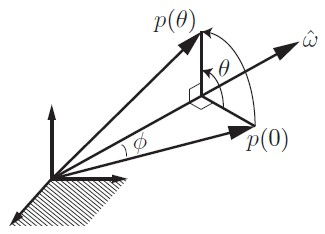考虑上图，给定旋转轴 ω ^ \hat{\omega} ，以及一个点 p p ，现将其从 p ( 0 ) p(0) 位置运动到 p ( θ ) p(\theta) 位置。假设旋转过程中速度为单位速度 1 r a d / s 1 rad/s ，那么该运动路径上任意一个点为 p ( t ) , t ∈ [ 0 , θ ] p(t),t\in[0,\theta] 。此时，如上图，计算其任意时刻速度为： p ˙ = ω ^ × p = ω ^ ∧ p \dot{p} = \hat{\omega} \times p = \hat{\omega}^{\wedge}p 结合其初始条件 p ( 0 ) p(0) ，我们可知
p ( t ) = e ω ^ ∧ t p ( 0 ) p(t) = e^{\hat{\omega}^{\wedge}t}p(0) 注意上述分析都是在单位速度假设下的。同样的，因为我们可以替换 t t θ \theta ，所以， p ( θ ) = e ω ^ ∧ θ p ( 0 ) p(\theta) = e^{\hat{\omega}^{\wedge}\theta}p(0)
若将 e ω ^ ∧ θ e^{\hat{\omega}^{\wedge}\theta} 进行Taylor展开，我们还可以得到 R o t ( ω ^ , θ ) = e ω ^ ∧ θ = I + sin ⁡ ( θ ) ω ^ ∧ + ( 1 − cos ⁡ ( θ ) ) ( ω ^ ∧ ) 2 ∈ S O ( 3 ) Rot(\hat{\omega},\theta) = e^{\hat{\omega}^{\wedge}\theta} = I + \sin(\theta)\hat{\omega}^{\wedge} + (1-\cos(\theta))(\hat{\omega}^{\wedge})^{2} \in SO(3) 这被称为 Rodrigues’ formula。

最后，如果使用 ω ^ θ \hat{\omega}\theta 来表示旋转矩阵 R R 的指数坐标，那么，反对称矩阵 ω ^ ∧ θ \hat{\omega}^{\wedge}\theta 就是旋转矩阵 R R 矩阵对数。顾名思义，矩阵对数是矩阵指数的逆：
exp ⁡ : ω ^ ∧ θ ∈ s o ( 3 ) → R ∈ S O ( 3 ) log ⁡ : R ∈ S O ( 3 ) → ω ^ ∧ θ ∈ s o ( 3 ) \begin{aligned} \exp:\quad & \hat{\omega}^{\wedge}\theta\in so(3) \to R \in SO(3) \\ \log:\quad & R \in SO(3) \to \hat{\omega}^{\wedge}\theta \in so(3) \end{aligned}
相比较矩阵指数的计算，矩阵对数计算相对复杂。给定旋转矩阵 R R ，找到旋转角度 θ ∈ [ 0 , π ] \theta\in[0,\pi] 以及单位旋转轴 ω ^ ∈ R 3 , ∥ ω ^ ∥ = 1 \hat{\omega}\in\mathbb{R}^{3}, \|\hat{\omega}\| = 1 使得 e ω ^ ∧ θ = R e^{\hat{\omega}^{\wedge}\theta} = R ， 此时须分三种情况讨论：

1. R = I R = I ，此时 θ = 0 \theta = 0 ω ^ \hat{\omega} 无定义，因为并没有发生旋转。
2. t r a c e ( R ) = − 1 trace(R) = -1 ，此时 θ = π \theta = \pi ω ^ \hat{\omega} 可以是下列任意一个向量： ω ^ = 1 2 ( 1 + r 33 ) [ r 13 r 23 1 + r 33 ]  or  1 2 ( 1 + r 22 ) [ r 12 1 + r 22 r 32 ]  or  1 2 ( 1 + r 11 ) [ 1 + r 11 r 21 r 31 ] \hat{\omega} = \frac{1}{\sqrt{2(1+r_{33})}}\begin{bmatrix} r_{13} \\ r_{23} \\ 1+r_{33} \end{bmatrix} \text{ or } \frac{1}{\sqrt{2(1+r_{22})}}\begin{bmatrix} r_{12} \\ 1+r_{22} \\ r_{32} \end{bmatrix} \text{ or } \frac{1}{\sqrt{2(1+r_{11})}}\begin{bmatrix} 1+r_{11} \\ r_{21} \\ r_{31} \end{bmatrix}
3. 其它情况下， θ = arccos ⁡ ( t r a c e ( R ) − 1 2 ) ∈ [ 0 , π ) \theta = \arccos\left( \frac{trace(R)-1}{2} \right)\in[0,\pi) ω ^ ∧ = 1 2 sin ⁡ θ ( R − R ⊤ ) \hat{\omega}^{\wedge} = \frac{1}{2\sin\theta}(R - R^{\top})

我们可以认为，李群 S O ( 3 ) SO(3) 是空间种一个球心在原点处，半径为 π \pi 的实心球体，如下图所示。任意点到球心的距离表示旋转的角度 θ \theta ，那么，当 θ = π \theta = \pi （即 t r a c e ( R ) = − 1 trace(R) = -1 ）时，球面上两个对极点表示同一个旋转。

## 空间刚体运动与Twist

上述李群与李代数的讨论仅用于研究旋转过程，下面我们将其进一步拓展到任意位移。简单地说，下述内容中的齐次变换矩阵 T T 可类比旋转矩阵 R R ，螺旋（screw）运动轴 S \mathcal{S} 可类比旋转轴 ω ^ \hat{\omega} ，而Twist V \mathcal{V} 可表示成 S θ ˙ \mathcal{S}\dot{\theta} 的形式，该形式类比角速度 ω ^ θ ˙ \hat{\omega}\dot{\theta} ，同理，刚体运动的指数坐标 S θ ∈ R 6 \mathcal{S}\theta \in \mathbb{R}^{6} 可类比旋转的指数坐标 ω ^ θ ∈ R 3 \hat{\omega}\theta\in\mathbb{R}^{3}

### Twist理论入门

关于齐次（Homogeneous）变换与齐次坐标的讨论此处从略，直接切入正题。我们同时考虑一个刚体的角速度与线速度。考虑如下齐次变换：
T s b ( t ) ≜ T ( t ) = [ R ( t ) p ( t ) 0 1 ] T_{sb}(t) \triangleq T(t) = \begin{bmatrix} R(t) & p(t) \\ 0 & 1 \end{bmatrix} 回顾旋转矩阵（李群）与李代数的关系，我们做类似的推导：
T − 1 T ˙ = [ R ⊤ − R ⊤ p 0 1 ] [ R ˙ p ˙ 0 0 ] = [ R ⊤ R ˙ R ⊤ p ˙ 0 0 ] = [ ω b ∧ v b 0 0 ] T^{-1}\dot{T} = \begin{bmatrix} R^{\top} & -R^{\top}p \\ 0 & 1 \end{bmatrix}\begin{bmatrix} \dot{R} & \dot{p} \\ 0 & 0 \end{bmatrix} = \begin{bmatrix} R^{\top}\dot{R} & R^{\top}\dot{p} \\ 0 & 0 \end{bmatrix} = \begin{bmatrix} \omega_{b}^{\wedge} & v_{b} \\ 0 & 0 \end{bmatrix} 注意 p ˙ \dot{p} 是刚体坐标系（动坐标系）在固定坐标系下的线速度，而 v b v_{b} 是该线速度表示在刚体坐标系下的。鉴于此表示，我们定义body twist（或刚体坐标系下的空间速度）为 V b = [ ω b v b ] ∈ R 6 , V b ∧ = [ ω b ∧ v b 0 0 ] ∈ s e ( 3 ) \mathcal{V}_{b} = \begin{bmatrix} \omega_{b} \\ v_{b} \end{bmatrix} \in \mathbb{R}^{6}, \quad \mathcal{V}_{b}^{\wedge} = \begin{bmatrix} \omega_{b}^{\wedge} & v_{b} \\ 0 & 0 \end{bmatrix}\in se(3) 即刚体坐标系 (Moving frame) 的线速度和角速度在一个与该刚体坐标系重合的静止坐标系下的表示。因此我们将 ω b \omega_{b} v b v_{b} 合成一个6维度的速度向量 V b \mathcal{V}_{b}
同样的， T ˙ T − 1 = [ R ˙ p ˙ 0 0 ] [ R ⊤ − R ⊤ p 0 1 ] = [ R ˙ R ⊤ p ˙ − R ˙ R ⊤ p 0 0 ] = [ ω s ∧ v s 0 0 ] \dot{T}T^{-1} = \begin{bmatrix} \dot{R} & \dot{p} \\ 0 & 0 \end{bmatrix}\begin{bmatrix} R^{\top} & -R^{\top}p \\ 0 & 1 \end{bmatrix} = \begin{bmatrix} \dot{R}R^{\top} & \dot{p}-\dot{R}R^{\top}p \\ 0 & 0 \end{bmatrix} = \begin{bmatrix} \omega_{s}^{\wedge} & v_{s} \\ 0 & 0 \end{bmatrix} 其中 ω s \omega_{s} 是该角速度在固定坐标系下的表示。
注意，有点特殊的是 v s = p ˙ − R ˙ R ⊤ p v_{s} = \dot{p}-\dot{R}R^{\top}p 并不等于刚体坐标系原点在固定坐标系下的线速度（ p ˙ \dot{p} ），我们稍作推导易知 v s = p ˙ − ω s × p = p ˙ + ω s × ( − p ) v_{s} = \dot{p} - \omega_{s}\times p = \dot{p} + \omega_{s}\times (-p) 也就是说 v s v_{s} 实际上是刚体运动在固定坐标系原点产生的实时线速度，如下图所示。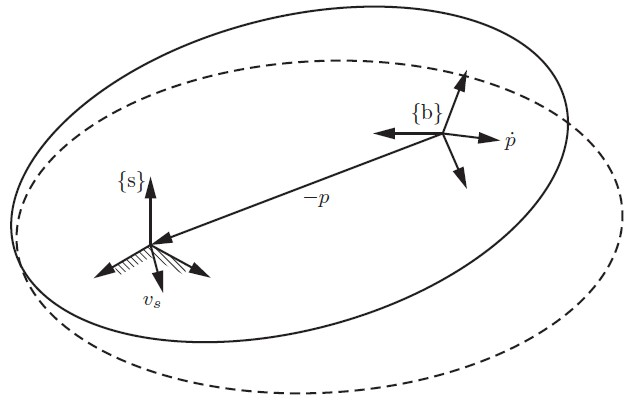我们定义spatial twist（或固定（空间）坐标系下的空间速度）为
V s = [ ω s v s ] ∈ R 6 , V s ∧ = [ ω s ∧ v s 0 0 ] ∈ s e ( 3 ) \mathcal{V}_{s} = \begin{bmatrix} \omega_{s} \\ v_{s} \end{bmatrix} \in \mathbb{R}^{6}, \quad \mathcal{V}_{s}^{\wedge} = \begin{bmatrix} \omega_{s}^{\wedge} & v_{s} \\ 0 & 0 \end{bmatrix}\in se(3) 类比李代数的坐标系变换，我们同样可以得到
V b ∧ = T − 1 V s ∧ T , V s ∧ = T V b ∧ T − 1 \mathcal{V}_{b}^{\wedge} = T^{-1}\mathcal{V}_{s}^{\wedge}T, \quad \mathcal{V}_{s}^{\wedge} = T\mathcal{V}_{b}^{\wedge}T^{-1}
[ ω s v s ] = [ R 0 p ∧ R R ] [ ω b v b ] \begin{bmatrix} \omega_{s} \\ v_{s} \end{bmatrix} = \begin{bmatrix} R & 0 \\ p^{\wedge}R & R \end{bmatrix}\begin{bmatrix} \omega_{b} \\ v_{b} \end{bmatrix} 相关推导十分简单直接，此处从略。这个变换十分有用，被称作伴随表示（adjoint representation），即给定 T = ( R , p ) ∈ S E ( 3 ) T = (R,p)\in SE(3) ，那么其伴随表示 [ A d T ] [\mathrm{Ad}_{T}] [ A d T ] = [ R 0 p ∧ R R ] ∈ R 6 × 6 [\mathrm{Ad}_{T}] = \begin{bmatrix} R & 0 \\ p^{\wedge}R & R \end{bmatrix} \in \mathbb{R}^{6\times 6}
伴随表示有如下特性
[ A d T 1 ] [ A d T 2 ] V = [ A d T 1 T 2 ] V [ A d T 1 ] − 1 = [ A d T 1 − 1 ] [ A d T 1 − 1 ] [ A d T 1 ] V = V \begin{aligned} [\mathrm{Ad}_{T_{1}}] [\mathrm{Ad}_{T_{2}}]\mathcal{V} = [\mathrm{Ad}_{T_{1}T_{2}}]\mathcal{V} \\ [\mathrm{Ad}_{T_{1}}]^{-1} = [\mathrm{Ad}_{T_{1}^{-1}}] \\ [\mathrm{Ad}_{T_{1}^{-1}}][\mathrm{Ad}_{T_{1}}]\mathcal{V} = \mathcal{V} \end{aligned}
与李代数类似 V s \mathcal{V}_{s} 的取值与刚体坐标系的选取无关， V b \mathcal{V}_{b} 的取值与固定坐标系的选取无关。值得注意的是，假设运动的刚体无限大，body twist V b \mathcal{V}_{b} 和 spatial twist V s \mathcal{V}_{s} 之间存在对称关系：

• ω b \omega_{b} 是刚体在刚体坐标系 { b } \{b\} 下的角速度， ω s \omega_{s} 是刚体在空间坐标系 { s } \{s\} 下的角速度。
• v b v_{b} 是刚体上一点在刚体坐标系 { b } \{b\} 下的线速度， v s v_{s} 是该刚体在空间原点处的点在空间坐标系 { s } \{s\} 下的线速度。

### Twist 的螺旋解读

类比角速度 ω \omega ，其可被看作是 ω ^ θ ˙ \hat{\omega}\dot{\theta} ；对于Twist，我们可以将其看作是一个螺旋轴 S \mathcal{S} 和绕着该轴的速度 θ ˙ \dot{\theta} 。关于螺旋运动的详细讨论此处从略，仅介绍关键部分。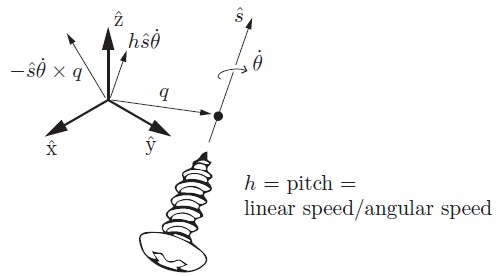如上图所示，给定旋转轴 s ^ \hat{s} 、旋转速度 θ ˙ \dot{\theta} 、旋转轴上任意点 q q screw pitch h h ，一个螺旋轴可表示为 S ≜ { q , s ^ , h } \mathcal{S} \triangleq \{q,\hat{s}, h\} 。其与Twist的关系是
V = [ ω v ] = [ s ^ θ ˙ − s ^ θ ˙ × q + h s ^ θ ˙ ] \mathcal{V} = \begin{bmatrix} \omega \\ v \end{bmatrix} = \begin{bmatrix} \hat{s}\dot{\theta} \\ -\hat{s}\dot{\theta} \times q + h\hat{s}\dot{\theta} \end{bmatrix} ω = 0 \omega = 0 时， h h 为无穷大。螺旋轴可以由Twist直接定义，

• ω ≠ 0 \omega\neq 0 时， S = V / ∥ ω ∥ = ( ω / ∥ ω ∥ , v / ∥ ω ∥ ) \mathcal{S} = \mathcal{V}/\|\omega\| = (\omega/\|\omega\|, v/\|\omega\|) .
• ω = 0 \omega = 0 时， S = V / ∥ v ∥ = ( 0 , v / ∥ v ∥ ) \mathcal{S} = \mathcal{V}/\|v\| = (0, v/\|v\|) .

其中 h = ω ⊤ v ∥ ω ∥ 2 h = \frac{\omega^{\top}v}{\|\omega\|^{2}} Screw 与 Twist 的相互关系在参考文献2第2章第3节3.3中有详细讨论。
螺旋轴 S \mathcal{S} 可被看作是单位长度的Twist，我们同样可以定义： S ∧ = [ ω ∧ v 0 0 ] ∈ s e ( 3 ) \mathcal{S}^{\wedge} = \begin{bmatrix} \omega^{\wedge} & v \\ 0 & 0 \end{bmatrix} \in se(3)
利用伴随矩阵，一个定义在参考系 { a } \{a\} 下的螺旋轴 S a \mathcal{S}_{a} 在参考系 { b } \{b\} 下可表示为： S a = [ A d T a b ] S b , S b = [ A d T b a ] S a \mathcal{S}_{a} = \left[\mathrm{Ad}_{T_{ab}}\right]\mathcal{S}_{b}, \quad \mathcal{S}_{b} = \left[\mathrm{Ad}_{T_{ba}}\right]\mathcal{S}_{a}

### 空间刚体运动的指数坐标表示

类比纯旋转变换的指数坐标 ω ^ θ \hat{\omega}\theta ，我们定义齐次变换的指数坐标 S θ \mathcal{S}\theta 。其中 S \mathcal{S} 是螺旋轴， θ \theta 是旋转距离。对于任意一个齐次变换（运动），如果 h h 是有限的，那么 ∥ ω ∥ = 1 \|\omega\|=1 θ \theta 是绕着该轴的旋转角度；如果 h h 是无穷大的，那么 ω = 0 \omega = 0 v = 1 v = 1 θ \theta 是沿着该轴的平移运动大小。

• exp ⁡ : S ∧ θ ∈ s e ( 3 ) → T ∈ S E ( 3 ) \exp: \mathcal{S}^{\wedge}\theta \in se(3) \to T \in SE(3) .
• log ⁡ : T ∈ S E ( 3 ) → S ∧ θ ∈ s e ( 3 ) \log: T\in SE(3) \to \mathcal{S}^{\wedge}\theta \in se(3) .

更一般的计算公式为（具体推导从略，同样是Taylor展开）：

• 对于 ∥ ω ∥ = 1 \|\omega\| = 1 的情形：
e S ∧ θ = [ e ω ∧ θ G ( θ ) v 0 1 ] e^{\mathcal{S}^{\wedge}\theta} = \begin{bmatrix} e^{\omega^{\wedge}\theta} & G(\theta)v \\ 0 & 1 \end{bmatrix} 其中 G ( θ ) ≜ I θ + ( 1 − cos ⁡ θ ) ω ∧ + ( θ − sin ⁡ θ ) ( ω ∧ ) 2 G(\theta) \triangleq I\theta+(1-\cos\theta)\omega^{\wedge}+(\theta-\sin\theta)(\omega^{\wedge})^{2} ；或者上式可以写成 e S ∧ θ = [ e ω ∧ θ ( I − e ω ∧ θ ) ( ω × v ) + ω ω ⊤ v θ 0 1 ] e^{\mathcal{S}^{\wedge}\theta} = \begin{bmatrix} e^{\omega^{\wedge}\theta} & (I-e^{\omega^{\wedge}\theta})(\omega \times v)+\omega\omega^{\top} v\theta \\ 0 & 1 \end{bmatrix} 事实上，考虑螺旋轴 { q , s ^ , h } \{q,\hat{s},h\} ，该运动可以写成： e S ∧ θ = [ e ω ∧ θ ( I − e ω ∧ θ ) q + h θ ω 0 1 ] e^{\mathcal{S}^{\wedge}\theta} = \begin{bmatrix} e^{\omega^{\wedge}\theta} & (I-e^{\omega^{\wedge}\theta})q+h\theta\omega \\ 0 & 1 \end{bmatrix} 该写法与上一个等价（ v = − ω × q + h ω v = -\omega\times q + h\omega ）。
• 对于 ω = 0 \omega = 0 ∥ v ∥ = 1 \|v\| = 1 的情形：
e S ∧ θ = [ I v θ 0 1 ] e^{\mathcal{S}^{\wedge}\theta} = \begin{bmatrix} I & v\theta \\ 0 & 1 \end{bmatrix}

需要注意的是对 e S ∧ θ e^{\mathcal{S}^{\wedge}\theta} 的解读：尽管该变换属于特殊欧式群（ S E ( 3 ) SE(3) ），但是它并非表示某一点 p p 从一个坐标系到另一个坐标系的映射关系，而是该点在从初始坐标 p ( 0 ) p(0) 到刚体运动后的对应点，即 p ( θ ) = e S ∧ θ p ( 0 ) p(\theta) = e^{\mathcal{S}^{\wedge}\theta}p(0) 其中 p ( 0 ) p(0) p ( θ ) p(\theta) 均是在同一个坐标系下的表示。这与空间位姿的齐次坐标表示的含义是不同的。同理，令 g a b ( θ ) ∈ S E ( 3 ) g_{ab}(\theta)\in SE(3) 表示刚体某时刻的位姿，那么 g a b ( θ ) = e S ∧ θ g a b ( 0 ) g_{ab}(\theta) = e^{\mathcal{S}^{\wedge}\theta}g_{ab}(0)

当然，给定一个齐次变换 T T 也可以求出相应的指数坐标，这便是对数运算。与纯旋转的情况类似，此处我们须分情况讨论。给定 T ∈ S E ( 3 ) T\in SE(3) ，那么

1. R = I R=I ，此时可令 ω = 0 \omega = 0 , v = p / ∥ p ∥ v = p/\|p\| θ = ∥ p ∥ \theta = \|p\| ，即纯平移变换。
2. t r a c e ( R ) = − 1 trace(R) = -1 ，此时 θ = π \theta = \pi ω \omega 的求解参考上一节内容； v = G − 1 ( θ ) p v = G^{-1}(\theta)p G − 1 ( θ ) = 1 θ I − 1 2 ω ∧ + ( 1 θ − 1 2 cot ⁡ θ 2 ) ( ω ∧ ) 2 G^{-1}(\theta) = \frac{1}{\theta}I - \frac{1}{2}\omega^{\wedge} + \left( \frac{1}{\theta} - \frac{1}{2}\cot\frac{\theta}{2} \right)(\omega^{\wedge})^{2}
3. 其它情况下， θ = arccos ⁡ ( t r a c e ( R ) − 1 2 ) ∈ [ 0 , π ) ω ∧ = 1 2 sin ⁡ θ ( R − R ⊤ ) v = G − 1 ( θ ) p \begin{aligned} \theta &= \arccos\left( \frac{trace(R) - 1}{2} \right)\in[0,\pi) \\ \omega^{\wedge} &= \frac{1}{2\sin\theta}(R-R^{\top}) \\ v &= G^{-1}(\theta)p \end{aligned}

需要注意的是，此时 ω \omega v v 都是单位向量，但是在Twist的定义中并不要求 ω \omega v v 是单位向量。

## 力、力矩与Wrench如上图，考虑作用于点 r r 上的线性力 f f ，假设以坐标系 { a } \{a\} 为参考，我们可以计算该点处的力矩（torque或moment）为 m a = r a × f a m_{a} = r_{a} \times f_{a} 。参考Twist的定义，我们定义 Wrench 为： F a = [ m a f a ] ∈ R 6 \mathcal{F}_{a} = \begin{bmatrix} m_{a} \\ f_{a} \end{bmatrix} \in \mathbb{R}^{6} 如果作用于该点处有多个力，那么其合Wrench的计算只需要将每个力产生的Wrench叠加即可，注意务必保证在一个参考系下。具有零线性部分的Wrench代表纯力矩（pure moment）。

不同参考系下Wrench向量的变换同样可借助伴随实现 F b = [ A d T a b ] ⊤ F a F a = [ A d T b a ] ⊤ F b \begin{aligned} \mathcal{F}_{b} &= [\mathrm{Ad}_{T_{ab}}]^{\top}\mathcal{F}_{a} \\ \mathcal{F}_{a} &= [\mathrm{Ad}_{T_{ba}}]^{\top}\mathcal{F}_{b} \end{aligned} 具体推导从略，参考功率与坐标系无关的特性 V b ⊤ F b = V a ⊤ F a \mathcal{V}_{b}^{\top}\mathcal{F}_{b} = \mathcal{V}_{a}^{\top}\mathcal{F}_{a}用一个例子来简述Wrench的用法，考虑上图所示夹持动作 { f } \{f\} 为末端力传感中心坐标系， { h } \{h\} 为夹持器中心坐标系， { a } \{a\} 为物体质心。假设夹持器重力为 5 N 5N ，物体重力为 1 N 1N 。此时，夹持器重点产生的Wrench为 F h = [ 0 , 0 , 0 , 0 , − 5 N , 0 ] ⊤ \mathcal{F}_{h} = [0, 0, 0, 0, -5N, 0]^{\top} 物体重点产生的Wrench 为 F a = [ 0 , 0 , 0 , 0 , 0 , 1 N ] ⊤ \mathcal{F}_{a} = [0,0,0,0,0, 1N]^{\top} 方便起见，将二者均定义在自身的坐标系下。若 L 1 = 0.1 m L_{1} = 0.1m L 2 = 0.15 m L_{2} = 0.15m ，那么 T h f = [ 1 0 0 − 0.1 0 1 0 0 0 0 1 0 0 0 0 1 ] , T a f = [ 1 0 0 − 0.25 0 0 1 0 0 − 1 0 0 0 0 0 1 ] T_{hf} = \begin{bmatrix} 1 & 0 & 0 & -0.1 \\ 0 & 1 & 0 & 0 \\ 0 & 0 & 1 & 0 \\ 0 & 0 & 0 & 1 \end{bmatrix}, \quad T_{af} = \begin{bmatrix} 1 & 0 & 0 & -0.25 \\ 0 & 0 & 1 & 0 \\ 0 & -1 & 0 & 0 \\ 0 & 0 & 0 & 1 \end{bmatrix} 那么，作用于坐标系 { f } \{f\} 原点处的合Wrench为： F f = [ A d T h f ] ⊤ F h + [ A d T a f ] ⊤ F a = [ 0 , 0 , − 0.5 , 0 , − 5 , 0 ] ⊤ + [ 0 , 0 , − 0.25 , 0 , − 1 , 0 ] ⊤ = [ 0 , 0 , − 0.75 , 0 , − 6 , 0 ] ⊤ \begin{aligned} \mathcal{F}_{f} &= [\mathrm{Ad}_{T_{hf}}]^{\top}\mathcal{F}_{h} + [\mathrm{Ad}_{T_{af}}]^{\top}\mathcal{F}_{a} \\ &= [0,0,-0.5,0,-5,0]^{\top} + [0,0,-0.25,0,-1,0]^{\top} \\ &=[0,0,-0.75,0,-6,0]^{\top} \end{aligned}

参考

1. Modern Robotics Mechanics Planning and Control. K. M. Lynch and F. C. Park, 2016.
2. A Mathematical Introduction to Robotic Manipulation. R. Murray, Z. Li and S. Sastry, 2005.
3. State Estimation for Robotics. T. D. Barfoot, 2017.
展开全文• Unity开发中直接使用Rigidbody.velocity属性来获取刚体的当前速度， 注意：Rigidbody.velocity 的陷阱，参考链接：https://blog.csdn.net/qq_34247835/article/details/81023383 具体工具代码： public static ...
• 理解起来，就是先找到该刚体，然后获取刚体对象，设置刚体线性速度，赋值给刚体自身。 如果不是初始化onload的时候设置刚体线性速度，建议用下面这种方式： this.RigidBody.linearVelocity = cc.p(500 * ...
• 无论刚体 质量 有多大，下落速度都一样。 （下落速度应该修改 重力大小 ）unity
• 问题遇到的现象和发生背景 在3D里，一个全新的实体添加了刚体，默认启用重力，会下坠，速度较快。我的游戏实体，装载了组件，默认启用重力，下坠速度却没有前者快了。 问题相关代码，请勿粘贴截图 using System....unity
• 算法思路在大地坐标系中，刚体上任意点A的位置可以下式表示： R=R0+r(1)R=R_0+r \tag 1 其中R0R_0表示刚体上的基点O在大地坐标系的位置， rr表示向量OA−→−\overrightarrow{OA}在大地坐标系中的坐标。 假设刚体...
• 移动看似是最简单的逻辑，但其实涉及知识面范围很广，且许多无论多花哨的游戏其良好体验的内核都是控制的手感...unity
• 刚体作定轴转动时，轴不转，轴个点的速度、加速度恒为0；轴以外个点均作圆周运动：圆心：在轴线圆周所在平面：与轴线垂直圆的半径R：等于该点到转轴的距离圆周运动的角速度、角加速度刚体定轴转动的角速度ω、...
• 用瞬心法求平面运动刚体上各点的加速度用瞬心法求平面运动刚体上各点的加速度用瞬心法求平面运动刚体上各点的加速度
• 快速点匹配算法，香港理工大学zhanglei副教授，进行非刚体匹配时，速度很快，本人强烈推荐
• 1.引言 线加速度计的测量值包含了 加速度计在某瞬时姿态下 重力加速度分量 和 运动加速度。在控制系统当中，我们常常需要获得刚体...这时可以借助安装在刚体上的编码器，来获得线加速度计的姿态角，也是可以减掉的。但
• 模拟小球从斜面滑到水平面上，因为斜着下降的速度有点慢所以把Physics.gravity = new Vector3(0, -300, 0);全局引力调大了，这样的话小球到水平面上的时候速度会突然下降。
• 刚体平面运动时,应用质点系对动点的动量矩定理,得出纯滚动的刚体和平面运动均质杆对速度瞬心的动量矩定理,应用其求解动力学问题比较简捷。
• pcd文件点云数据刚体变换，可绕x,y,z轴旋转一定角度 + 平移 运行速度
• ## 刚体

千次阅读 2018-05-22 15:56:11
刚体是一种为了方便物理计算而提出的理想化模型，在不需要考虑物体本身的形变时，可以把物体当做刚体进行处理。 在 Unity 中，提供了 Rigidbody 组件，用来模拟刚体，我们叫它刚体组件。给游戏对象添加刚体组件，就...Unity 小波
• Vector3 localVelocity = transform... 如何只获取刚体其中一个方向速度 transform.InverseTransformDirection(rigidbody.velocity.z) 转载于:https://www.cnblogs.com/feiyanstudio/articles/7326764.html
• 我通过施加水平力，改变水平速度，来实现2d人物左右移动，但是竖直方向的速度也会随之改变 ，弹力和摩擦力都设为0了 ，而且这个y速度只显示却不影响竖直方向的移动,像是凭空产生的一样。但是我想通过判断y方向的速度...unity
• ## 刚体与非刚体

千次阅读 2021-11-27 16:46:38
从物理学的角度，刚体就是在受力或者运动时，形状和大小不变，而且内部各点的相对位置不变的物体。 刚体运动没有形变，没有变形速度。 非刚体运动则有，包括线变形速率和角变形速率。 ...机器学习 python
• 做了个导弹的模型,需要设置加力发射或者速度发射,所以整理了下代码备忘 if (isAddForceLaunch) {//加力发射 GetComponent().relativeForce = new Vector2(0, StartForce); } else {//速度发射 float ...unity3D velocity Vector2
• ;刚体的质量是通过碰撞组件的 密度 与 大小 自动...有些时候可能会希望获取刚体上某个点的移动速度比如一个盒子旋转着往前飞碰到了墙这时候可能会希望获取盒子在发生碰撞的点的速度可以通过 getLinearVelocityFromWorl
• 以椭圆规尺和曲柄连杆的平面运动为例，通过运用Maple建立相应的运动模型，讨论了刚体上各点速度和加速度之间的关系。研究结果表明：Maple是一种高性能的可视化计算机软件，它可使教学中复杂和难以讲解的问题简单化，...
• 为了简化振动筛的刚体平面运动的力学数学模型,利用曲柄连杆将其...求出了转动筛的速度、加速度瞬心,结合质心速度、加速度,给出了转动筛任意点速度、加速度的求解方法,为振动筛设计及物料运动的分析提出了一种简单方法。
• 【Unity】11.2 刚体（Rigidbody） 分类：Unity、C#、VS2015 创建日期：2016-05-02 一、简介 Rigidbody（刚体）组件可使游戏对象在物理系统的控制下来运动，刚体可接受外力与扭矩力，使游戏对象像在真实世界中那样...unity学习 unity刚体
• 研究了两个刚体角速度运动关于部分状态变量——惯性主轴角速度的同步控制。基于李亚普诺夫部分稳定性理论，分别采用双向耦合的部分状态变量线性反馈控制和部分变量的单向非线性反馈控制两种方法，构造出了一些控制器......